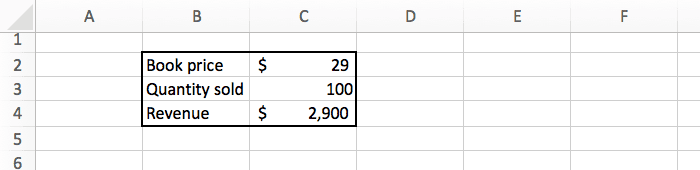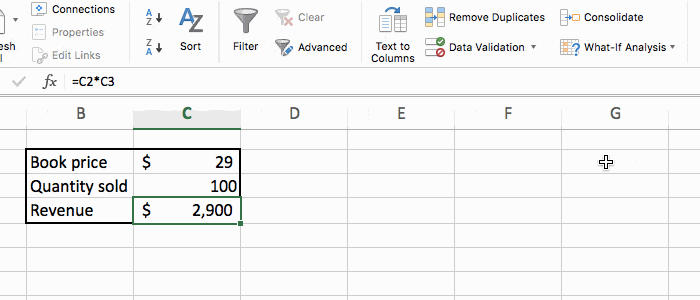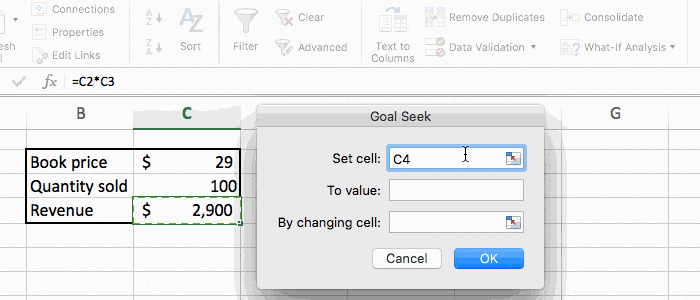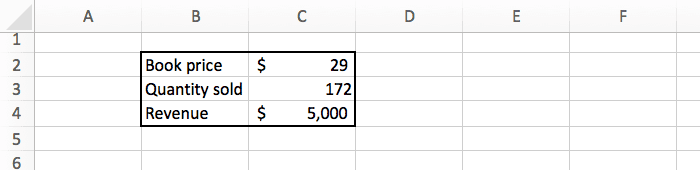# Excel: The What If Analysis With Goal Seek

There are some Microsoft Excel features that are awesome but somewhat hidden. And the "goal seek analysis" is one of them.

Sometimes you'll have a formula with a specific goal in mind, like "I want the result to be exactly 42". When you don't know how to change the formula's input to get that number, you should use goal seek.

## The Dataset

To use the goal seek feature we will need some data first.

Here's a table with 2 inputs (book price and quantity sold), and a formula (revenue = price * quantity).If we sell 100 books at \$29 each, we will make \$2,900.

Let's say we want to get exactly \$5,000 in revenue from the book sales. How many books should we sell to get that result? There are 3 ways to answer that question:

• Do some math and figure out the answer by yourself.
• Try different values of "quantity sold" manually in Excel until you find the correct answer.
• Use goal seek to get the result almost instantaneously.

Since this is a tutorial about goal seek, let's see how to do the last option ;-)

## Goal Seek Example

Select the cell containing the revenue, and in the "data" tab click on the "what if analysis" button. There select the "goal seek" option.A popup will appear that you need to fill like this:

• Set cell: the cell we want to set, that's the "revenue" in C4. This field should already have C4 in it.
• To value: our goal, which is 5000.
• By changing: the input we want to change, that's the "quantity sold" in C3.Press OK, and we are done! Excel did the math for us, and automatically updated the spreadsheet with the correct answer. In this case we see that we need to sell 172 copies to get \$5,000 in revenue.Actually the number in the cell is 172.41, but it is rounded in the screenshot above.

## Conclusion

With just a few clicks, we can get Excel to do some magic computation and give us interesting information.

For this example we had a simple formula. But you could do the same on something much more complex, and Excel will give you the perfect answer in no time!